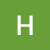# Solving Popular Algorithms: Add Strings

`let arr1 = num1.split("")let arr2 = num2.split("")let numZs = 0  if (num1.length > num2.length) {    numZs = num1.length - num2.length    while (numZs > 0) {      arr2.unshift("0")      numZs--    }  } else {    numZs = num2.length - num1.length    while (numZs > 0) {      arr1.unshift("0")      numZs--    }}`
`let results = []let secondDig = 0for (let i = arr1.length-1; i >= 0; i--) {    let sumNum = parseInt(arr1[i]) + parseInt(arr2[i])   if (sumNum >= 10) {       arr1[i-1] = (parseInt(arr1[i-1]) + 1).toString()      let gettingSecondDig = sumNum.toString().split("")      secondDig = gettingSecondDig      if (i === 0) {          results.unshift(sumNum.toString())      } else {          results.unshift(secondDig)      }       } else {      results.unshift(sumNum.toString())   }}`
`addStrings = (num1, num2) => {   let arr1 = num1.split("")   let arr2 = num2.split("")   let numZs = 0   if (num1.length > num2.length) {   numZs = num1.length - num2.length   while (numZs > 0) {      arr2.unshift("0")      numZs--    }   } else {    numZs = num2.length - num1.length    while (numZs > 0) {      arr1.unshift("0")      numZs--    }  }      let results = []   let secondDig = 0   for (let i = arr1.length-1; i >= 0; i--) {      let sumNum = parseInt(arr1[i]) + parseInt(arr2[i])      if (sumNum >= 10) {          arr1[i-1] = (parseInt(arr1[i-1]) + 1).toString()         let gettingSecondDig = sumNum.toString().split("")         secondDig = gettingSecondDig         if (i === 0) {            results.unshift(sumNum.toString())         } else {            results.unshift(secondDig)         }          } else {         results.unshift(sumNum.toString())      }   }      return results.join("")}`

--

--

--

## More from HopeGiometti

Love podcasts or audiobooks? Learn on the go with our new app.

## A nitty gritty details about Node.js’s modules & packages## How Single Page Applications Broke Web Design## 10 React Basic Fundamentals Concept.## How Create Chatbot for Website using jQuery## Laravel’s URL::to() vs URL::asset()## Redux Data Flow## 4 Practical Use Cases for IIFEs in JavaScript## Angular (v4) + Typescript Easy REST## Why do array indexes mostly start at 0?## Aiming for object-oriented design elegance## Blind 75 Javascript Edition — Dynamic Programming (Part 2)## Solve: Merge Sorted Array